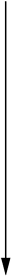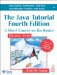# Expressions, Statements, and Blocks

Variables and operators, which you met in the previous two sections, are basic building blocks of programs. You combine literals, variables, and operators to form expressionssegments of code that perform computations and return values. Certain expressions can be made into statementscomplete units of execution. By grouping statements together with braces{ and }you create blocks of code.

This section covers expressions, statements, and blocks. The next section discusses a kind of statement, called a control flow statement, that affects the flow of your program.

Expressions

Expressions perform the work of a program. Among other things, expressions are used to compute and to assign values to variables and to help control the execution flow of a program. The job of an expression is twofold: to perform the computation indicated by the elements of the expression and to return a value that is the result of the computation.

Definition

An expression is a series of variables, operators, and method calls (constructed according to the syntax of the language) that evaluates to a single value.

As discussed in the previous section, operators return a value, so the use of an operator is an expression. This partial listing of the MaxVariablesDemo program shows some of the program's expressions in boldface:

```// other primitive types
char aChar = 'S';
boolean aBoolean = true;

// display them all
System.out.println("The largest byte value is " + largestByte);
...
if (Character.isUpperCase(aChar)) {
...
} ```

Each expression performs an operation and returns a value, as shown in Table 19.

Table 19. Expressions in the MaxVariablesDemo Program

Expression

Action

Value Returned

aChar = 'S'

Assign the character 'S' to the character variable aChar

The value of aChar after the assignment ('S')

"The largest byte value is " + largestByte

Concatenate the string "The largest byte value is " and the value of largestByte converted to a string

The resulting string: The largest byte value is 127

Character.isUpperCase(aChar)

Call the method isUpperCase

The return value of the method: true

The data type of the value returned by an expression depends on the elements used in the expression. The expression aChar = 'S' returns a character because the assignment operator returns a value of the same data type as its operands, and aChar and 'S' are characters. As you see from the other expressions, an expression can return a boolean value, a string, and so on.

The Java programming language allows you to construct compound expressions from various smaller expressions as long as the data type required by one part of the expression matches the data type of the other. Here's an example of a compound expression:

`x * y * z `

In this particular example, the order in which the expression is evaluated is unimportant because the results of multiplication are independent of order; the outcome is always the same, no matter what order you apply the multiplications. However, this is not true of all expressions. For example, the following expression gives different results, depending on whether you perform the addition or the division operation first:

`x + y / 100 //ambiguous `

You can specify exactly how you want an expression to be evaluated, using balanced parentheses( and ). For example, to make the previous expression unambiguous, you could write:

`(x + y) / 100 //unambiguous, recommended `

If you don't explicitly indicate the order in which you want the operations in a compound expression to be performed, the order is determined by the precedence assigned to the operators in use within the expression. Operators with a higher precedence get evaluated first. For example, the division operator has a higher precedence than does the addition operator.

Thus, the two following statements are equivalent:

```x + y / 100
x + (y / 100) //unambigous, recommended ```

When writing compound expressions, you should be explicit and indicate with parentheses which operators should be evaluated first. This will make your code easier to read and to maintain.

Table 20 shows the precedence assigned to the operators in the Java platform. The operators in this table are listed in precedence order: The higher in the table an operator appears, the higher its precedence. Operators with higher precedence are evaluated before operators with a relatively lower precedence. Operators on the same line have equal precedence.

 Highest Precedence Postfix operators [] . (params ) expr ++ expr --Unary operators ++expr --expr +expr -expr ~ ! Creation or cast new (type )expr Multiplicative * / % Additive + - Shift > >>> Relational < > <= >= instanceof Equality == != Bitwise AND & Bitwise exclusive OR ^ Bitwise inclusive OR | Conditional AND && Conditional OR || Shortcut if-else ?: Lowest Precedence Assignment = += -= *= /= %= &= ^= |= <<= >>= >>>=

When operators of equal precedence appear in the same expression, a rule must govern which is evaluated first. All binary operators except for the assignment operators are evaluated from left to right. Assignment operators are evaluated right to left.

Statements

Statements are roughly equivalent to sentences in natural languages. A statement forms a complete unit of execution. The following types of expressions can be made into a statement by terminating the expression with a semicolon (;):

• Assignment expressions
• Any use of ++ or --
• Method calls
• Object creation expressions

These kinds of statements are called expression statements. Here are some examples of expression statements:

```aValue = 8933.234; //assignment statement
aValue++; //increment statement
System.out.println(aValue); //method call statement
Integer integerObject = new Integer(4); //object creation statement ```

In addition to these kinds of expression statements, there are two other kinds of statements. A declaration statement declares a variable. You've seen many examples of declaration statements.

`double aValue = 8933.234; // declaration statement `

A control flow statement regulates the order in which statements get executed. The for loop and the if statement are both examples of control flow statements. You'll learn about control flow statements in the section Control Flow Statements (page 99).

Blocks

A block is a group of zero or more statements between balanced braces and can be used anywhere a single statement is allowed. The following listing shows two blocks from the MaxVariablesDemo program, each containing a single statement:

```if (Character.isUpperCase(aChar)) {
System.out.println("The character " + aChar + " is upper case.");
} else {
System.out.println("The character " + aChar + " is lower case.");
} ```

Summary of Expressions, Statements, and Blocks

An expression is a series of variables, operators, and method calls (constructed according to the syntax of the language) that evaluates to a single value. You can write compound expressions by combining expressions as long as the types required by all the operators involved in the compound expression are correct. When writing compound expressions, you should be explicit and indicate with parentheses which operators should be evaluated first.

If you choose not to use parentheses in a compound expression, the Java platform evaluates it in the order dictated by operator precedence. Table 20 (page 96) shows the relative precedence assigned to the operators in the Java platform.

A statement forms a complete unit of execution and is terminated with a semicolon (;). There are three kinds of statements: expression statements, declaration statements, and control flow statements.

You can group zero or more statements together into a block with braces: { and }. Even though not required, we recommend using blocks with control flow statements even if only one statement is in the block.

Questions and Exercises: Expressions, Statements, and Blocks

Questions

 1: What are the data types of the following expressions, assuming that i's type is int? ```i > 0 i = 0 i++ (float)i i == 0 "aString" + i ``` 2: Consider the following expression: `i--%5>0 ` What is the result of the expression, assuming that the value of i is initially 10? Modify the expression so that it has the same result but is easier for programmers to read.

Exercises

 1: Write a program to confirm your answers to questions 2a and 2b.

You can find answers to these Questions and Exercises online: